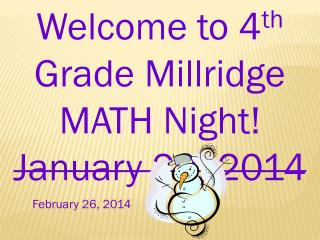DownloadDownload PresentationWelcome to 4 th Grade Millridge MATH Night! January 29, 2014

# Welcome to 4 th Grade Millridge MATH Night! January 29, 2014

Télécharger la présentation## Welcome to 4 th Grade Millridge MATH Night! January 29, 2014

- - - - - - - - - - - - - - - - - - - - - - - - - - - E N D - - - - - - - - - - - - - - - - - - - - - - - - - - -
##### Presentation Transcript

1. Welcome to 4th Grade Millridge MATH Night! January 29, 2014 February 26, 2014

2. Common Core 4.NF.A.1 Explain why a fraction is equal to another using visual models EX: Stella & Brian each heat up a small pizza. Stella cuts hers into 4 equal slices. Brian cuts his into 8 equal slices. Draw models of Stella’s & Brian’s pizza. Now Stella eats 2 pieces of her pizza & Brian eats 4 pieces. Do they eat the same amount of pizza? Explain using the models.

3. Common core 4NF.A.2 Compare 2 fractions with different numerators & denominators by creating common denominators or by comparing to a benchmark fraction like ½ EX: A kitchen floor has green, yellow & white tiles. Of those, 2/5 are green and 2/10 are yellow. Are there more green tiles than yellow tiles? Explain how you know this.

4. Common core 4NF.B.3a. Add & subtract fractions with like denominators EX: Amy walks 1/5 of a mile on Monday and on Tuesday she walks 2/5 of a mile. How far did she walk total in the 2 days?

5. Common core 4.NF.B.3b. Decompose a fraction into a sum of fractions with the same denominator EX: Chloe used fraction strips to show 7/8 as the sum of fractions. Draw another way to show 7/8 as the sum of fractions. 1

6. Common core 4.NF.B.3c. Add & subtract mixed numbers with like denominators Add: 1 ¼ + + 2 2/4 ____________________________ 3 ¾

7. Common core 4.NF.B.3d. Solve word problems involving adding & subtracting fractions. EX: George is mixing colors to paint a door. He pours 3/8 cup of red paint into a can. Then he pours 2/8 cup of blue paint into the can. How much paint is in the can now?

8. Common core 4.NF.B.4a Understand a fraction as a multiple EX: Julie divides a board into 8 equal pieces. Draw a model to show Julie’s board. Julie paints 3/8 of the board. How many pieces of the board does she paint? How many pieces of the board are not painted?

9. Common core 4.NF.B.4b. Multiply a fraction by a whole number EX: 4 x ¾ = explain how you know answer: 4 x 3 x ¼ = 12/4 = 3 Multiply 4/7 and 2= answer: 4 x 2 x 1/7 = 8/7 or 1 1/7

10. Common core 4.NF.B.4c solve word problems involving multiplying of a fraction by a whole number. EX: Fern is putting lace around napkins. For each napkin, she needs 3/5 yds. of lace. Fern will put lace around 10 napkins. Write an equation to solve this and explain how many yards of lace Fern will need total.

11. Common core 4.NF.C.5 Express a fraction with a denominator of 10 as equal to a fraction with a denominator of 100 EX: 4/10 = 40/100 EX: 4/10 + 3/100 = _____

12. Common core 4.NF.C.6 Find equal fractions to decimals with denominators of 10 & 100 EX: 4/10 = 0.4 22/100 = 0.22 Common core 4.NF.C.7 Compare decimals EX: 0.43 > 0.39 0.2 = 0.20